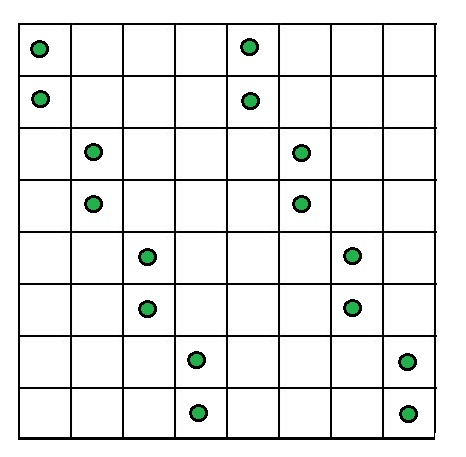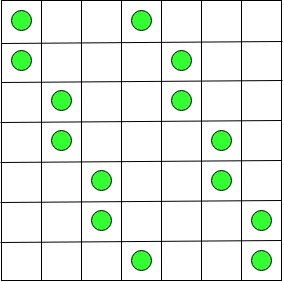# Puzzle | The Counters and Board

Given 2n counters where n > 1. You have to place these 2n counters on an n × n board so that no more than
two counters are in the same row, column, or diagonal.

Solution :
Since we need to place 2n counters into n rows and n columns of the given n x n board, with the condition that there can be at most two counters in the same row or in the same column. From this , we can conclude that exactly two counters have to be placed in each row and column.

Case 1 : For even n = 2k, we can obtain a solution by identical placement of n counters in the first k columns and the last k columns as explained below.
Let us assume that rows and columns of the board are numbered top to bottom and left to right, respectively.
Place two counters in the first two rows of columns 1 and k + 1, two counters in rows 3 and 4 of columns 2 and k + 2, and and so on, until finally counters are placed in rows n – 1 and n of columns k and 2k. Let us understand this with the help of an example when n = 8 :2n Counters problem for n = 8

Case 2 : For odd n = 2k + 1, k > 0, we can obtain a solution by placing two counters in rows 1 and 2 of column 1, two counters in rows 3 and 4 of column 2, and so on until counters are placed in rows n – 2 and n – 1 of column k. Then two counters are placed in the first and last rows of column k + 1. After that, k counters are placed in the right part of the board symmetrically with respect to the board’s central square to those in the left part: two counters are placed in rows 2 and 3 of column k + 2, in rows 4 and 5 of column k + 3, and so on, until rows n – 1 and n of the last column.Let us understand this with the help of an example when n = 7 :2n countern problem for n = 7

My Personal Notes arrow_drop_upIn love with a semicolon because sometimes i miss it so badly)

If you like GeeksforGeeks and would like to contribute, you can also write an article using contribute.geeksforgeeks.org or mail your article to contribute@geeksforgeeks.org. See your article appearing on the GeeksforGeeks main page and help other Geeks.

Please Improve this article if you find anything incorrect by clicking on the "Improve Article" button below.

Improved By : HiteshSaini

Article Tags :
Practice Tags :

1

Please write to us at contribute@geeksforgeeks.org to report any issue with the above content.